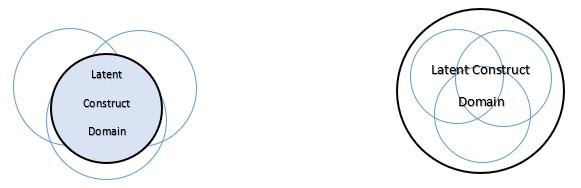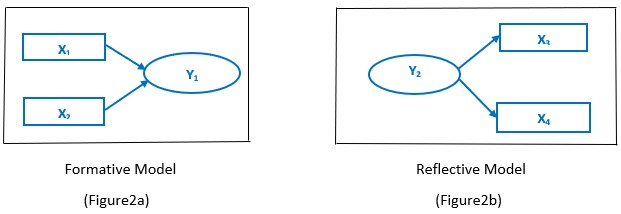# Cross-eyed PhD: Tip 11 SEM Modeling part 2

posted in: Wealth of learning | 0
###### Piled High and Deep”, www.PHDCOMICS.com, by Jorge Cham

Editor’s Note: This post by contributing author, Sanjay Malla, Research Scholar develops the discussion on SEM with a brief on the “Formative and Reflective” measurement Models. It is important for PhD Scholars to realize that rigorous statistical modeling is not beyond the scope of their research!

## Beginners guide to Structure Equation Modeling (SEM) Part – II

### Types of Measurement Models

In our previous article on SEM, we focused on Structural Equation Modeling using partial least square path modeling technique and went on to explain how researchers build and  visually display the hypotheses and variable relationships. There are two sub-models in a structured equation model; first, there is an inner model that represents the relationships between the independent and dependent latent variables. Second, there is outer model that specifies the relationships between the constructs and their observed indicators.

In this series, we will focus on outer model (also known as Measurement model) and explain how the latent variables (constructs) are measured in different ways. There are two types of measurement scales in SEM;

#### Reflective and Formative Measurement Scales

The reflective model (also referred as Mode-A measurement) is based on classical theory. According to his theory, measures represent the manifestations of a latent construct therefore causality is from the construct to its measures (Figure2b). Reflective indicators can be perceived as a representative sample of all the possible indicators within the theoretical domain of the latent construct.##### (Figure1a)                                                                                 (Figure1b)

Since a reflective measure suggests that all the indicator items are caused by the same construct, indicators related to a particular construct are highly correlated with each other and therefore interchangeable. Any single indicator can be dropped without actually altering the true nature of the construct, as long as the construct maintains sufficient reliability. Since the causal relationship goes from the construct to its measures, it should be noted that any change in the construct will cause simultaneous change in the indicators.The formative model (also referred to Mode-B measurement) is based on the assumption that the indicators cause the construct (Figure2a). In contrast to reflective model, the formative indicators are not interchangeable. Each indicator which helps in forming a construct captures a specific aspect of the construct’s domain. When analyzed collectively, these items explain the meaning of the construct, which means that by dropping an indicator would potentially change the very nature of the construct. Therefore, the width of coverage of the construct is important to ensure that the domain of content of the latent construct is adequately represented.

Figures (1a &1b) illustrate the key differences between the reflective and formative models. The black circles represent construct domain, which is the total content the construct is expected to measure. The blue circles represent the scope each indicator captures. The reflective model (Figure1a), aims to maximize the overlap between the interchangeable indicators, the formative model (Figure1b) aims to fully cover the construct domain by various indicators of small overlap.

It’s important to note that by default, PLS-SEM assumes the indicators are reflective when the model is initially built, with arrows pointing away from the construct. It’s therefore very important for researcher to change the direction of the arrows when the indicators are formative instead of reflective. In case you are not sure whether to measure a construct reflectively or formatively, the Confirmatory Tetrad Analysis (CTA-PLS) can be performed to figure it out quantitatively.

Note: Next article in this series shall evaluate PLS-SEM results using dummy data from a customer survey in a restaurant (B2C) segment. Output shall be generated & interpreted using ADANCO, PLS-SEM software package.

Sanjay Malla

Doctoral Scholar

Semiconductor & Embedded Systems Professional

….and here is a quote to guide your research…